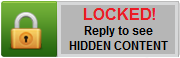Erchima Gaming Forums

Hack the game and enjoy playing it!

[TRAINER] Starpoint Gemini 2 Hack v3.1 +20 (Steam)Game process:
StarpointGemini2.exe
Hotkey and features:

• Numeric 1 = God mode
• Numeric 2 = Infinity Skill Energy
• Numeric 3 = Infinity Weapon Energy
• Numeric 4 = Infinity Shield
• Numeric 5 = Infinity Missiles
• Numeric 6 = Infinity Items
• Numeric 7 = Infinity T-Drive (only 1.5000)
• Numeric 8 = Infinity T-Drive (only 1.5000 SOA)
• Numeric 9 = Infinity T-Drive (only 1.5000 Origins)
• Numeric 0 = Player status
• CTRL + Numeric 1 = Current Level
• CTRL + Numeric 2 = Current Reputation
• CTRL + Numeric 3 = Current EXP
• CTRL + Numeric 4 = Current Money
• CTRL + Numeric 5 = Current Skill Points
• CTRL + Numeric 6 = Ship stats
• CTRL + Numeric 7 = Current Cargo
• CTRL + Numeric 8 = Max Cargo
• CTRL + Numeric 9 = Current Troops
• CTRL + Numeric 0 = Max Troops

Require:

• Trainer
• Cheat Engine

Tutorial:

1. Open Starpoint Gemini 2 (StarpointGemini2.exe)
2. Open trainer
3. Play game for 1 min
4. Back to trainer
5. Press hotkey
6. Enjoy hacking